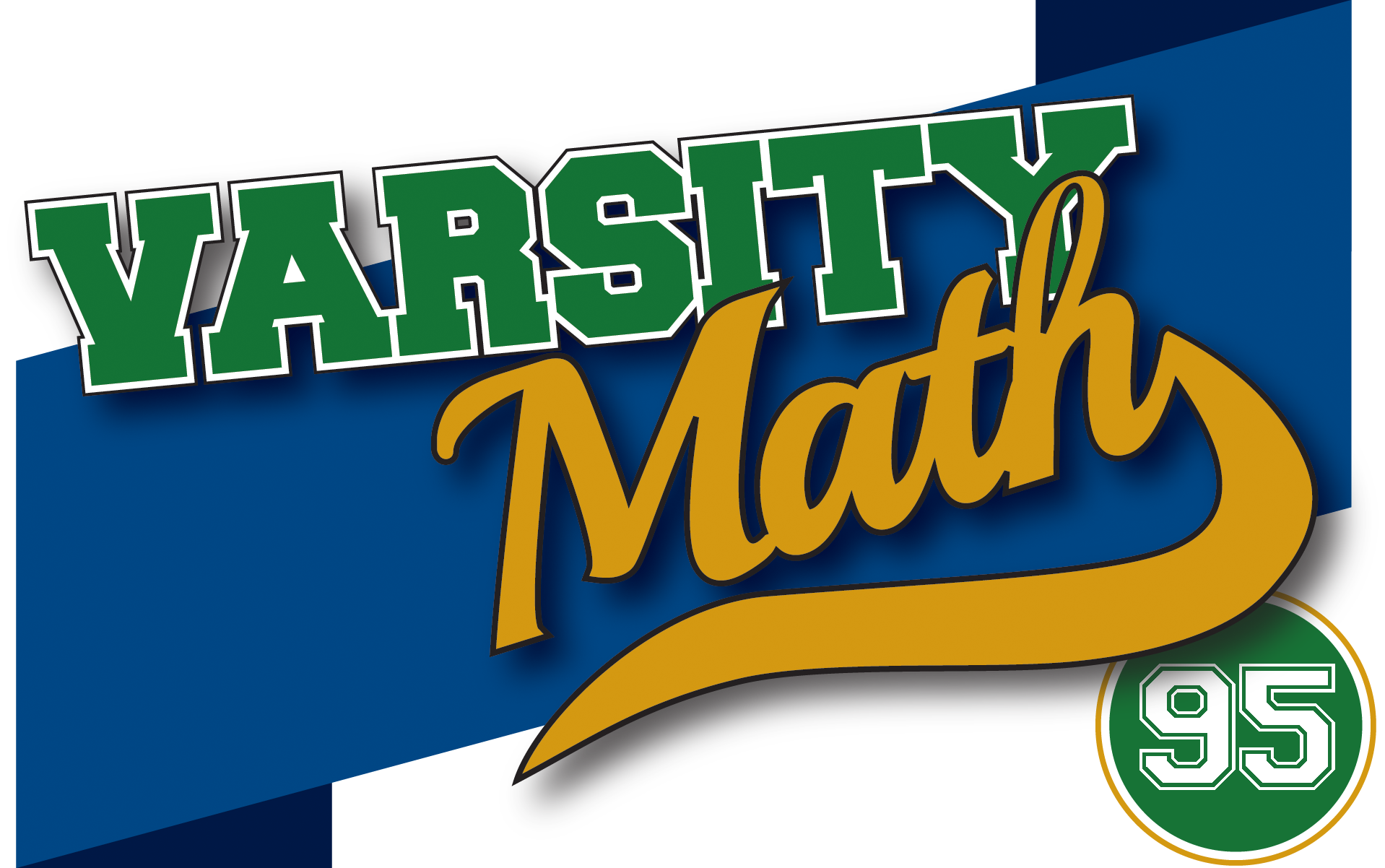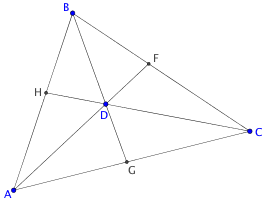## ________________

This week’s problems are based on a 2005 Math Horizons article written by the great mathematics and science writer Martin Gardner (1914-2010).

## ________________

### Proper Place

Consider all of the counting numbers squashed into one long string of digits, like so: 12345678910111213141516… The “proper place” of a number is the position that the first digit of its occurrence in counting order occupies in this string of digits. For example, the proper place of 13 is 16, because the “1” of the “13” occurs in the 16th position in this sequence of digits.

What is the proper place of 2017?

### Earliest BirdMartin Gardner called a number an “early bird” if it appears in the above sequence before its proper place. For example, twelve is an early bird because “12” appears as the first two digits of the sequence, even though its proper place is 14. In fact, it is exactly 13 places early; that’s its proper place minus the position of the first digit of its actual first occurrence in the sequence. On the other hand, some numbers like eleven are not early birds at all; their first occurrences are exactly in their proper places. As a warmup for this problem, you can figure out whether or not 2017 is an early bird.

What early bird less than or equal to 1,000 is early by the most places?

## Solutions to week 94

Penny Race. Note that once a T has occurred, if the first player didn’t win (i.e., the T did not occur after an HH), then the second player is guaranteed to win: any number of single Hs and groups of Ts might occur, but eventually there will be a double H, occurring after a T, and that is the second player’s pattern, and the first player can’t win until after that double H. So, if the first two coins come up HH the first player will win, but in the three remaining possibilities (HT, TH, and TT) the second player will go on to win. Hence the probability that the second player will win is 3/4.

Quirky Quads. In fact with just one more point,namely five points, there is guaranteed to be a convex quadrilateral. To see this, imagine each point is a nail in a board, and you stretch a giant rubber band large enough to surround all of the points and let go. It will close down tight around the “outermost” nails to create a polygon, and that polygon will always be convex, since the rubber band is stretchy and doesn’t “waste” any distance. In fact, that shape is called the convex hull of the points. If the convex hull touches all five points, then any four of them form a convex quadrilateral. If the convex hull touches four points, then that’s the desired convex quadrilateral. If it only touches three of them, then there is a triangle of the of the points ABC which contains the other two D and E. Draw lines from each of A,B,C through D until they reach the opposite sides at F,G,H as shown. If E is in AGD or BDF then quadrilateral BAED or BADE is convex. If E is in BHD or CDG then quadrilateral CBED or CBDE is convex. If E is in ADH or CFD then quadrilateral ACDE or ACED is convex. Since E is somewhere inside ABC, one of these possibilities must hold, so there is always a convex quadrilateral.